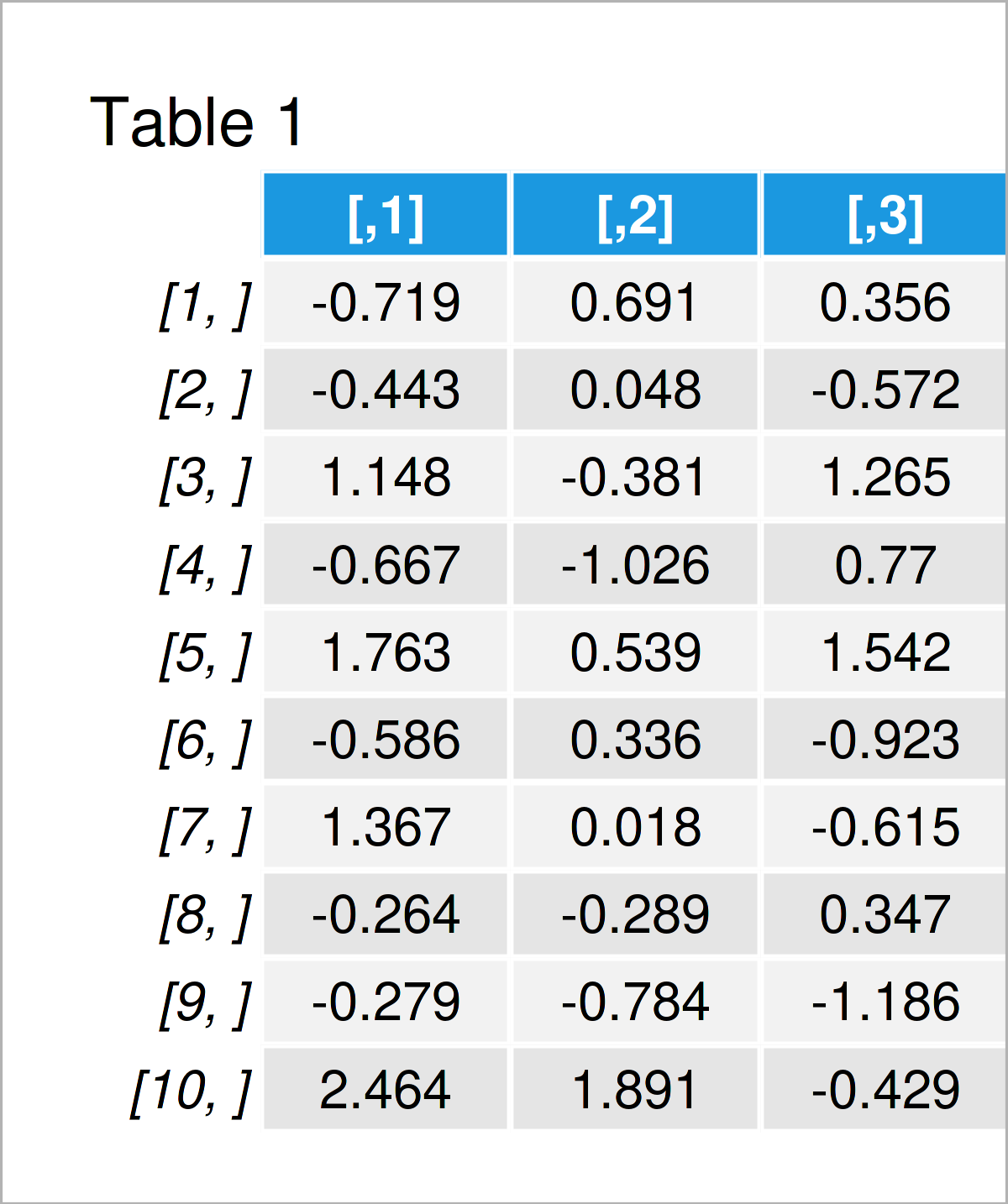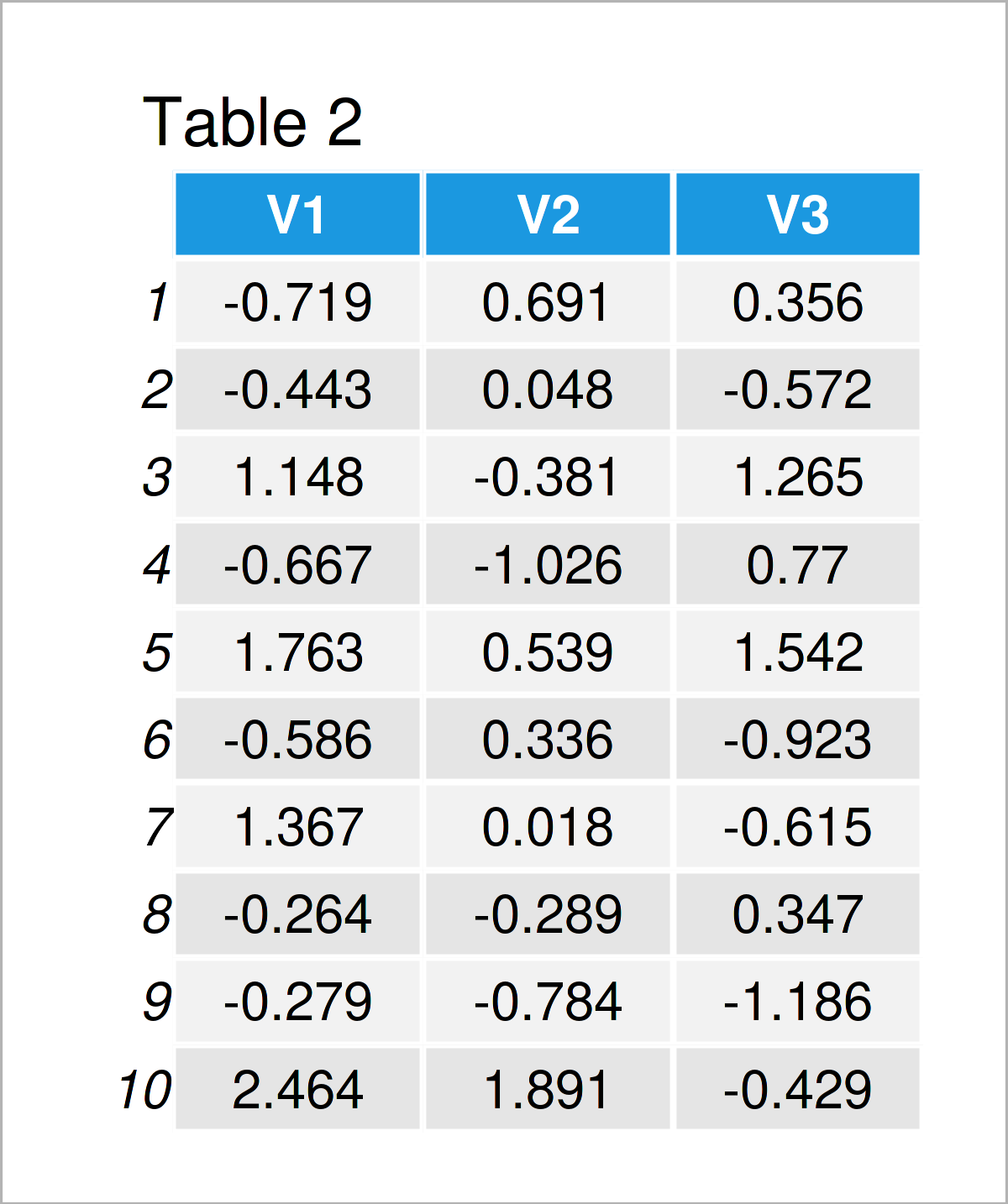# Generate Matrix with i.i.d. Normal Random Variables in R (2 Examples)

This tutorial explains how to create a data matrix containing independent and identically distributed (i.i.d.) normal random columns in R programming.

With that, let’s dive right in.

## Example 1: Generate Matrix with i.i.d. Normal Random Variables

Example 1 shows how to generate a matrix that contains multiple i.d.d. variables.

As a first step, we have to define the dimensions (i.e. the number of rows and columns) of our matrix:

```N_row <- 10                               # Define number of rows & columns
N_col <- 3```

Furthermore, we should set a random seed for reproducibility:

`set.seed(39825)                           # Set random seed`

In the next step, we can apply the matrix and rnorm functions to create an i.i.d. random matrix:

```my_mat <- matrix(rnorm(N_row * N_col),    # Create random matrix
ncol = N_col)
my_mat                                    # Print random matrix```By executing the previous R programming code, we have created Table 1, i.e. a matrix containing normally distributed random values.

## Example 2: Generate Data Frame with i.i.d. Normal Random Variables

In Example 2, I’ll demonstrate how to create a data frame object containing randomly distributed variables.

For this, we can use the matrix object that we have created in Example 1. To this matrix object, we can apply the as.data.frame function as shown below:

```my_df <- as.data.frame(my_mat)            # Create random data frame
my_df                                     # Print random data frame```After executing the previous R code the data frame shown in Table 2 has been created.

## Video, Further Resources & Summary

In case you need more explanations on the contents of this tutorial, you may have a look at the following video on my YouTube channel. In the video, I illustrate the R programming syntax of this article in RStudio.

Please accept YouTube cookies to play this video. By accepting you will be accessing content from YouTube, a service provided by an external third party.If you accept this notice, your choice will be saved and the page will refresh.

Furthermore, you may have a look at the related tutorials which I have published on this website. Some related articles are listed below.

This tutorial has shown how to construct a data matrix containing i.i.d. normal random variables in the R programming language. Let me know in the comments section, in case you have additional questions.

Subscribe to the Statistics Globe Newsletter